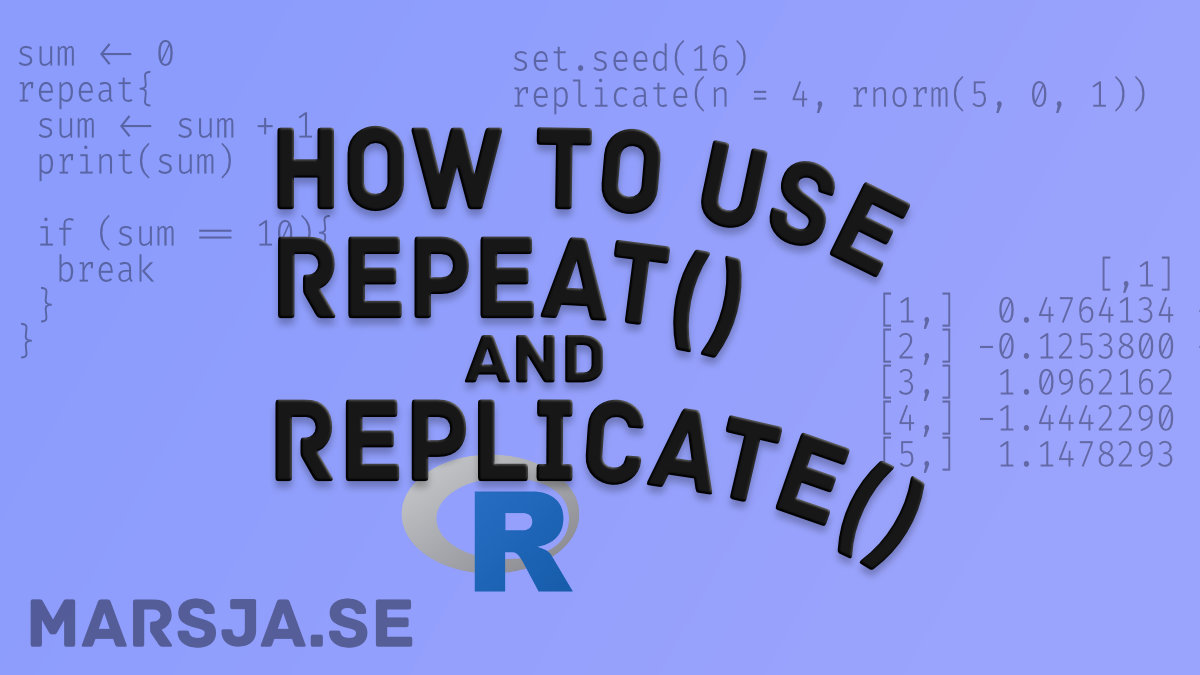17 Shares

In this short tutorial, we will learn how to use the repeat and replicate functions in R. These two functions, repeat and replicate, are two very useful functions.

## Repeat and Replicate

Now, before we learn how to use both repeat and replicate, we will answer two questions concerning them.

## What is the Repeat Function?

Now, what does the repeat() function in R actually do? The the repeat() function in R executes a same block of code iteratively until a condition we have set is met.

## What is the Replicate Function?

Replicate, on the other hand, is a wrapper for the common use of sapply for repeated evaluation of an expression (e.g., generating random numbers).

## Repeat in R

In this section, we will learn about the repeat() function in R. First, we’ll learn about the general syntax of the function, and then how to use it by example.

Note, we must put a condition inside the body of the loop and use the break statement to exit the loop. If we don’t do this we will have an infinite loop (see image above).

### Syntax of the Repeat Function

We can use the repeat function, in R, according to the following template:

```.wp-block-code {
border: 0;
}

.wp-block-code > div {
overflow: auto;
}

.shcb-language {
border: 0;
clip: rect(1px, 1px, 1px, 1px);
-webkit-clip-path: inset(50%);
clip-path: inset(50%);
height: 1px;
margin: -1px;
overflow: hidden;
position: absolute;
width: 1px;
word-wrap: normal;
word-break: normal;
}

.hljs {
box-sizing: border-box;
}

.hljs.shcb-code-table {
display: table;
width: 100%;
}

.hljs.shcb-code-table > .shcb-loc {
color: inherit;
display: table-row;
width: 100%;
}

.hljs.shcb-code-table .shcb-loc > span {
display: table-cell;
}

.wp-block-code code.hljs:not(.shcb-wrap-lines) {
white-space: pre;
}

.wp-block-code code.hljs.shcb-wrap-lines {
white-space: pre-wrap;
}

.hljs.shcb-line-numbers {
border-spacing: 0;
counter-reset: line;
}

.hljs.shcb-line-numbers > .shcb-loc {
counter-increment: line;
}

.hljs.shcb-line-numbers .shcb-loc > span {
}

.hljs.shcb-line-numbers .shcb-loc::before {
border-right: 1px solid #ddd;
content: counter(line);
display: table-cell;
text-align: right;
-webkit-user-select: none;
-moz-user-select: none;
-ms-user-select: none;
user-select: none;
white-space: nowrap;
width: 1%;
}
```repeat {
if(condition) {
break
}
}```Code language: R (r)```

Now, if we are working with dataframes, we might also need to remove a column in R. In the next section, we will learn how to use the repeat() function in R by example.

## How to use the repeat() function in R with an Example

Now, we are going to learn how to use the repeat function in R by an example. In the example below, we are not going to create a repeat loop that will go on forever. We will use the if statement together with the break function.

### Repeat in R Example

In this repeat() example we are running the repeat loop until sum becomes 10.

``````sum < 0
repeat{
sum < sum + 1
print(sum)

if (sum == 10){
break
}
}```Code language: R (r)```

Note, in the code chunk, above, we create the sum variable, in the repeat loop, we add one to the sum, print the sum, and the if-statement is controlling whether the sum is 10, or not, ever iteration of the repeat loop. Now, when sum is 10 R breaks out of the repeat loop.

If we need to import data we can learn all about reading xlsx files in R in an earlier post. In the next section, we will learn how to use the replicate() function by examples.

## How to use the replicate() function in R with Examples

In this section, we are going to learn how to use the replicate function in R.

Now, there are, of course, other useful functions in base R. For example, you can use the t() function to transpose a matrix or dataframe in R.

### A Simple Example of How to use replicate() in R

In this replicate in R example, we are going to simulate values from a normal distribution. This is accomplished by using the rnorm() function. In the simplest example, we can simulate 10 values, with a mean of 0, and a standard deviation of 1.

``````set.seed(2020)
rnorm(10, mean = 0, sd = 1)```Code language: R (r)```

Now, the above code gives us the following output:

As you can see in the image above, rnorm() produces a single set of simulated values. Now, you might wonder How do I simulate n values from this same distribution multiple times? Here’s exactly when the replicate() function in R can be really handy.

``````set.seed(2020)
replicate(n = 4, rnorm(5, 0, 1), simplify = FALSE))```Code language: R (r)```

Note, if we don’t use simplify = FALSE we would get a matrix. See the image below, where we have a 4 x 5 matrix with the values.

After we have simulated data in R using the replicate function together with rnorm, we might want to calculate some descriptive statistics using R and dplyr(). If the simulated data also contains categorical variables, learn how to create dummy variables in R.

## Conclusion: Repeat and Replicate in R

In this post, we have looked at the repeat loop and the replicate function in R. We learned repeat() need a condition (if-statement) and a break, or else we’ll get an infinite loop. Finally, we learned about replicate() and how to create a list or a matrix, from a replicated function.17 Shares